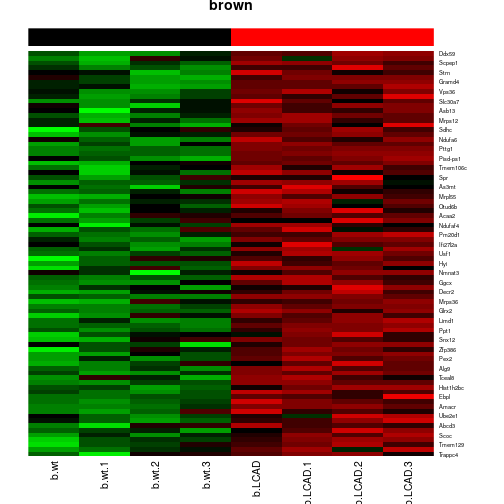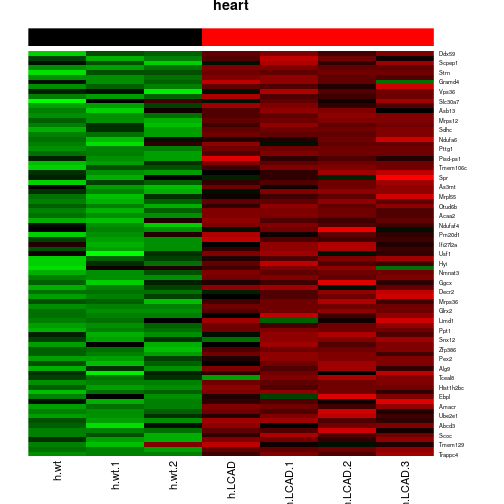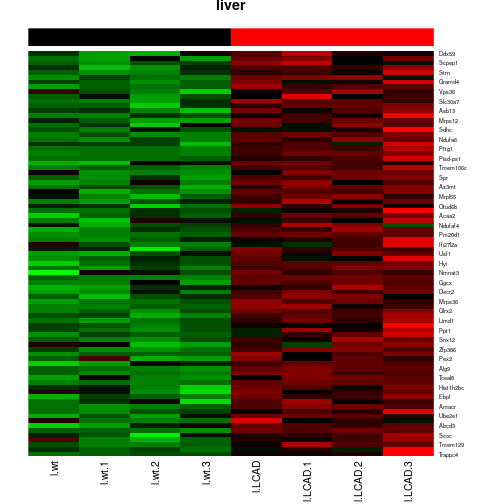# Introduction

## Background

Meta-analysis aims to combine summary statistics (e.g., effect sizes, p-values) from multiple clinical or genomic studies in order to enhance statistical power. Another appealing feature of meta-analysis is that batch effect (non-biological differences between studies because of sample platforms and experimental protocols) can be avoided, because the summary statistics are usually considered as standardized. The adaptively weighted Fisher's method (AW-Fisher) is an effective approach to combine $$p$$-values from $$K$$ independent studies and to provide better biological interpretability by characterizing which studies contribute to the meta-analysis.

## Statistical method

Denote $$\theta_k$$ is the effect size of study $$k$$, $$1\le k \le K$$). The AW-Fisher's method targets on biomarkers differentially expressed in one or more studies. The null hypothesis $$H_0$$ and the alternative hypothesis are listed below. $H_0: \vec{\boldsymbol{\theta}}\in \bigcap \{ \theta_k=0 \}$
$H_A: \vec{\boldsymbol{\theta}}\in \bigcup \{ \theta_k \ne 0 \},$

Define $$T(\vec{\textbf{P}}; \vec{\textbf{w}} ) = -2 \sum_{k=1}^K w_k \log P_k$$, where $$\vec{\textbf{w}} = (w_1, \ldots, w_K) \in {\{ 0,1 \} }^K$$ is the AW weight associated with $$K$$ studies and $$\vec{\textbf{P}} = (P_1, \ldots, P_K) \in {(0,1)}^K$$ is the random variable of input $$p$$-value vector for $$K$$ studies. The AW-Fisher's method will find the optimal weight $$\vec{\textbf{w}}^*$$, and calculate the test statistics and AW-Fisher p-value based on $$\vec{\textbf{w}}^*$$.

Collectively, the AW-Fisher's method will provide knowledge about which study contributes to the meta-analysis result via $$\vec{\textbf{w}}^*$$, and also generate p-value for rejecting the null hypothesis $$H_0$$.

This is a tutorial for the usage of the AWFisher package. A real data example of the multiple-tissue mouse metabolism data is used. The major contents of this tutorial includes:

• How to prepare the input for AWFisher.
• Transcriptomic meta analysis.
• Meta-analysis differential expression pattern (meta-pattern) detection.

## How to install the package

To install this package, start R (version “3.6”) and enter:

if (!requireNamespace("BiocManager", quietly = TRUE))
install.packages("BiocManager")

BiocManager::install("AWFisher")


## How to cite the package

• Huo, Z., Tang, S., Park, Y. and Tseng, G., 2020. P-value evaluation, variability index and biomarker categorization for adaptively weighted Fisher’s meta-analysis method in omics applications. Bioinformatics, 36(2), pp.524-532.

• The manuscript can be found here: https://www.ncbi.nlm.nih.gov/pubmed/31359040

## Maintainer

Zhiguang Huo (zhuo@ufl.edu)

## Description about the example data – multi-tissue mouse metabolism transcriptomic data

The purpose of the multi-tissue mouse metabolism transcriptomic data is to study how the gene expression changes with respect to the energy deficiency using mouse models. Very long-chain acyl-CoA dehydrogenase (VLCAD) deficiency was found to be associated with energy metabolism disorder in children. Two genotypes of the mouse model - wild type (VLCAD +/+) and VLCAD-deficient (VLCAD -/-) - were studied for three types of tissues (brown fat, liver, heart) with 3 to 4 mice in each genotype group. The sample size information is available in the table below. A total of 6,883 genes are available in this example dataset.

Brown Fat 4 4
Heart 3 4
Skeleton 4 4

## Read in the example data

library(AWFisher) # Include the AWFisher package

data(data_mouseMetabolism)

# Verify gene names match across three tissues
all(rownames(data_mouseMetabolism$brown) == rownames(data_mouseMetabolism$heart))
#>  TRUE
all(rownames(data_mouseMetabolism$brown) == rownames(data_mouseMetabolism$liver))
#>  TRUE

dataExp <- data_mouseMetabolism

# Check the dimension of the three studies
sapply(dataExp, dim)
#>      brown heart liver
#> [1,]  6883  6883  6883
#> [2,]     8     7     8

# Check the head of the three studies
#> $brown #> b.wt b.wt.1 b.wt.2 b.wt.3 b.LCAD b.LCAD.1 b.LCAD.2 #> Copg1 8.086841 8.047482 8.140015 8.010229 8.206645 8.151032 7.956093 #> Atp6v0d1 9.807054 9.637094 10.044481 9.825333 9.868880 9.667059 9.541244 #> b.LCAD.3 #> Copg1 8.086200 #> Atp6v0d1 9.581028 #> #>$heart
#> Copg1    7.859429 7.955171 8.045601 8.145281 8.016827 7.961778 7.964703
#> Atp6v0d1 9.479398 9.499617 9.571348 9.469063 9.516679 9.437079 9.559526
#>
#> $liver #> l.wt l.wt.1 l.wt.2 l.wt.3 l.LCAD l.LCAD.1 l.LCAD.2 #> Copg1 8.501327 8.698994 8.095882 8.519093 8.539002 8.305171 8.588183 #> Atp6v0d1 9.969806 9.975494 10.000650 10.161694 10.051711 10.084761 9.989209 #> l.LCAD.3 #> Copg1 8.554201 #> Atp6v0d1 10.035293 # Before performing differential expression analysis for each of these three tissues. # Create an empty matrix to store p-value. # Each row represents a gene and each column represent a study/tissue. pmatrix <- matrix(0,nrow=nrow(dataExp[]),ncol=length(dataExp)) rownames(pmatrix) <- rownames(dataExp[]) colnames(pmatrix) <- names(dataExp)  ## Prepare the input p-value matrix – perform differential expression analysis in each study library(limma) # Include the limma package to perform differential expression analyses for the microarray data for(s in 1:length(dataExp)){ adata <- dataExp[[s]] ControlLabel = grep('wt',colnames(adata)) caseLabel = grep('LCAD',colnames(adata)) label <- rep(NA, ncol(adata)) label[ControlLabel] = 0 label[caseLabel] = 1 design = model.matrix(~label) # design matrix fit <- lmFit(adata,design) # fit limma model fit <- eBayes(fit) pmatrix[,s] <- fit$p.value[,2]
}

#>              brown     heart     liver
#> Copg1    0.7148393 0.3554053 0.7586203
#> Atp6v0d1 0.1584368 0.7154922 0.8502931


# Perform AW Fisher meta analysis using the multi-tissue mouse metabolism transcriptomic data

res <- AWFisher_pvalue(pmatrix) ## Perform AW Fisehr meta analysis
qvalue <- p.adjust(res$pvalue, "BH") ## Perform BH correction to control for multiple comparison. sum(qvalue < 0.05) ## Differentially expressed genes with FDR 5% #>  755 head(res$weights) ## Show the AW weight of the first few genes
#>      [,1] [,2] [,3]
#> [1,]    0    1    0
#> [2,]    1    0    0
#> [3,]    1    0    1
#> [4,]    1    1    0
#> [5,]    1    1    1
#> [6,]    0    0    1


# Differential expression pattern (meta-pattern) detection.

## Calculate dissimilarity matrix

## prepare the data to feed function biomarkerCategorization
studies <- NULL
for(s in 1:length(dataExp)){
label[ControlLabel] = 0
label[caseLabel] = 1

}

## Set B = 1,000 (at least) for real data application
## You may need to wrap up a function (i.e., function_limma)
## to perform differential expression analysis for each study.

set.seed(15213)
result <- biomarkerCategorization(studies,function_limma,B=100,DEindex=NULL)
#> generate DE index since it is NULL
#> based on AW fdr  0.05
#> calculating permutated score, b = 1,2,..., B (= 100)  [one "." per sample]:
#> .................................................. 50
#> ...........................
#> Warning: Zero sample variances detected, have been offset away from zero
#> ....................... 100
#>
#>  calculating variability index
sum(result$DEindex) ## print out DE index at FDR 5% #>  755 head(result$varibility, n=2) ## print out the head of variability index
#>        [,1]   [,2]   [,3]
#> [1,] 0.7884 0.9424 0.9600
#> [2,] 0.6864 0.6400 0.7296
print(result$dissimilarity[1:4,1:4]) ## print out the dissimilarity matrix #> Psph Trappc4 Atg5 Cox18 #> Psph 1.00 0.00 0.01 0 #> Trappc4 0.00 1.00 0.75 0 #> Atg5 0.01 0.75 1.00 0 #> Cox18 0.00 0.00 0.00 1  ## Apply the tight clustering algorithm to get gene modules with unique meta-pattern library(tightClust) ## load tightClust package tightClustResult <- tight.clust(result$dissimilarity, target=4, k.min=15, random.seed=15213)
#> Number of points: 755    Dimension: 755
#>
#> Looking for tight cluster 1 ...
#> k = 15
#> k = 16
#> 1 tight cluster(s) found!
#> Cluster size: 85     Remaining number of points: 670
#>
#> Looking for tight cluster 2 ...
#> k = 14
#> k = 15
#> 2 tight cluster(s) found!
#> Cluster size: 66     Remaining number of points: 604
#>
#> Looking for tight cluster 3 ...
#> k = 13
#> k = 14
#> 3 tight cluster(s) found!
#> Cluster size: 65     Remaining number of points: 539
#>
#> Looking for tight cluster 4 ...
#> k = 12
#> k = 13
#> 4 tight cluster(s) found!
#> Cluster size: 64     Remaining number of points: 475
clusterMembership <- tightClustResult$cluster  ## Visualize the heatmap of the first meta-pattern module for all three tissues.  for(s in 1:length(dataExp)){ adata <- dataExp[[s]] aname <- names(dataExp)[s] bdata <- adata[qvalue<0.05, ][tightClustResult$cluster == 1 ,]
cdata <- as.matrix(bdata)
ddata <- t(scale(t(cdata))) # standardize the data such that for each gene, the mean is 0 and sd is 1.

B <- 16
redGreenColor <- rgb(c(rep(0, B), (0:B)/B), c((B:0)/16, rep(0, B)), rep(0, 2*B+1))
heatmap(ddata,Rowv=NA,ColSideColors=ColSideColors,col= redGreenColor ,scale='none',Colv=NA, main=aname)
}sessionInfo()
#> R version 3.6.2 (2019-12-12)
#> Platform: x86_64-pc-linux-gnu (64-bit)
#> Running under: Ubuntu 18.04.3 LTS
#>
#> Matrix products: default
#> BLAS:   /home/biocbuild/bbs-3.10-bioc/R/lib/libRblas.so
#> LAPACK: /home/biocbuild/bbs-3.10-bioc/R/lib/libRlapack.so
#>
#> locale:
#>   LC_CTYPE=en_US.UTF-8       LC_NUMERIC=C
#>   LC_TIME=en_US.UTF-8        LC_COLLATE=C
#>   LC_MONETARY=en_US.UTF-8    LC_MESSAGES=en_US.UTF-8
#>   LC_PAPER=en_US.UTF-8       LC_NAME=C
#>  LC_MEASUREMENT=en_US.UTF-8 LC_IDENTIFICATION=C
#>
#> attached base packages:
#>  stats     graphics  grDevices utils     datasets  methods   base
#>
#> other attached packages:
#>  tightClust_1.1 limma_3.42.2   AWFisher_1.0.1
#>
#> loaded via a namespace (and not attached):
#>   edgeR_3.28.0    compiler_3.6.2  magrittr_1.5    tools_3.6.2
#>   Rcpp_1.0.3      stringi_1.4.5   highr_0.8       grid_3.6.2
#>   locfit_1.5-9.1  knitr_1.28      stringr_1.4.0   xfun_0.12
#>  lattice_0.20-38 evaluate_0.14